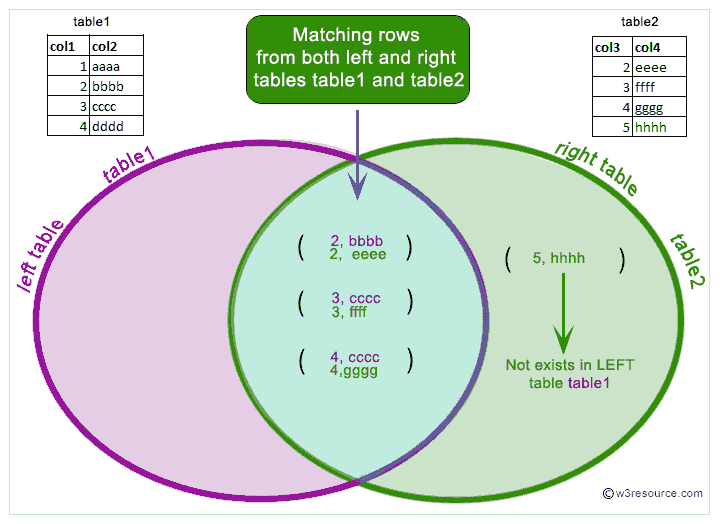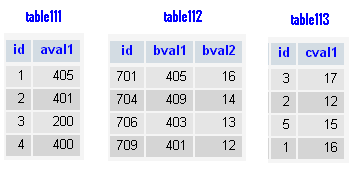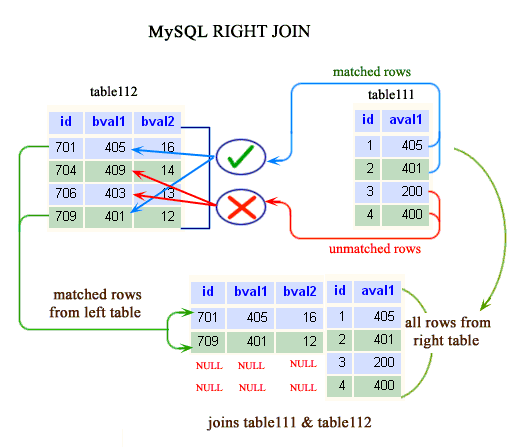# MySQL RIGHT JOIN

## What is RIGHT JOIN in MySQL?

The MySQL RIGHT JOIN joins two tables and fetches rows based on a condition, which is matching in both the tables and the unmatched rows will also be available from the table written after the JOIN clause.

Pictorial presentation of MySQL RIGHT JOIN:MySQL RIGHT JOIN Syntax:

MySQL supports the following JOIN syntaxes for the table_references (A table reference is also known as a join expression.) part of SELECT statements and multiple-table UPDATE and DELETE statements:

```table_references:
escaped_table_reference [, escaped_table_reference] ...

escaped_table_reference:
table_reference
| { OJ table_reference }

table_reference:
table_factor
| join_table

table_factor:
tbl_name [PARTITION (partition_names)]
[[AS] alias] [index_hint_list]
| table_subquery [AS] alias
| ( table_references )

join_table:
table_reference [INNER | CROSS] JOIN table_factor [join_condition]
| table_reference STRAIGHT_JOIN table_factor
| table_reference STRAIGHT_JOIN table_factor ON conditional_expr
| table_reference {LEFT|RIGHT} [OUTER] JOIN table_reference join_condition
| table_reference NATURAL [{LEFT|RIGHT} [OUTER]] JOIN table_factor

join_condition:
ON conditional_expr
| USING (column_list)

index_hint_list:
index_hint [, index_hint] ...

index_hint:
USE {INDEX|KEY}
[FOR {JOIN|ORDER BY|GROUP BY}] ([index_list])
| IGNORE {INDEX|KEY}
[FOR {JOIN|ORDER BY|GROUP BY}] (index_list)
| FORCE {INDEX|KEY}
[FOR {JOIN|ORDER BY|GROUP BY}] (index_list)

index_list:
index_name [, index_name] ...```

Suppose: 1st_table RIGHT JOIN 2nd_table JOIN CONDITION

In MySQL RIGHT JOIN, table 1st_table depends on table 2nd_table and all tables on which 2nd_table depends and also table 2nd_table depends on all tables that are used in the RIGHT JOIN condition except 1st_table. The RIGHT JOIN condition is used to decide how to retrieve rows from table 1st_table.

If there is a row in 2nd_table that matches the WHERE clause, but there is no row in 1st_table that matches the ON condition, an extra 1st_table row is generated with all columns set to NULL.

So, in case of RIGHT JOIN or RIGHT OUTER JOIN, MySQL -

1. takes all selected values from the right table

2. combines them with the column names ( specified in the condition ) from the left table

3. retrieve the matching rows from both the associated tables.

4. sets the value of every column from the left table to NULL which is unmatched with the right table.

Example: MySQL RIGHT JOIN

In the following example bval1 and aval1 of both the associated table have matched and all the specified columns for matching from both the table have appeared. The unmatched row from the right table i.e. table111 have appeared and for those rows, the columns of left table i.e. table112 have set a value NULL.

What happens here, MySQL starts with the Right table (table111). For each aval1 from the table table111 MySQL scans the left table - table112 to find the matching with bval1. When it finds the matching aval1 and bval1 it returns the other specified columns. For unmatched rows it returns null. Here, from the above example, it returns NULL for the value of aval1 200 and 400 because it does not exist in left table.

Code:

``````SELECT table112.id,table112.bval1,table112.bval2,
table111.id,table111.aval1
FROM table112
RIGHT JOIN table111
ON table112.bval1=table111.aval1;
```
```

Code:

``````SELECT table112.id,table112.bval1,table112.bval2,
table111.id,table111.aval1
FROM table112
RIGHT OUTER JOIN table111
ON table112.bval1=table111.aval1;
```
```

Sample tables:Sample Output:

```+------+-------+-------+------+-------+
| id   | bval1 | bval2 | id   | aval1 |
+------+-------+-------+------+-------+
|  701 |   405 |    16 |    1 |   405 |
|  709 |   401 |    12 |    2 |   401 |
| NULL |  NULL |  NULL |    3 |   200 |
| NULL |  NULL |  NULL |    4 |   400 |
+------+-------+-------+------+-------+
4 rows in set (0.00 sec)```

Pictorial PresentationMySQL RIGHT JOIN with USING clause

In the following example, a USING clause has used with RIGHT JOIN. The USING (column_list) clause names a list of columns that must exist in both tables.

Code:

``````SELECT table113.id,table113.cval1,
table111.id,table111.aval1
FROM table113
RIGHT OUTER JOIN table111
USING(id);
```
```

Sample Output:

```+------+-------+------+-------+
| id   | cval1 | id   | aval1 |
+------+-------+------+-------+
|    1 |    16 |    1 |   405 |
|    2 |    12 |    2 |   401 |
|    3 |    17 |    3 |   200 |
| NULL |  NULL |    4 |   400 |
+------+-------+------+-------+
4 rows in set (1.00 sec)```

Key points to remember

Click on the following to get the slides presentation -RIGHT JOIN: SQL and other Relational Databases

Previous: LEFT JOIN
Next: STRAIGHT_JOIN

﻿